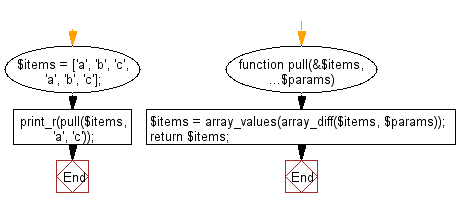﻿ PHP Exercise: Mutate the original array to filter out the values specified - w3resource

# PHP Exercises: Mutate the original array to filter out the values specified

## PHP: Exercise-88 with Solution

Write a PHP program to mutate the original array to filter out the values specified.

Sample Solution: -

PHP Code:

``````<?php
//Licence: https://bit.ly/2CFA5XY
function pull(&\$items, ...\$params)
{
\$items = array_values(array_diff(\$items, \$params));
return \$items;
}
\$items = ['a', 'b', 'c', 'a', 'b', 'c'];
print_r(pull(\$items, 'a', 'c'));

?>
```
```

Sample Output:

```Array
(
 => b
 => b
)```

Flowchart:PHP Code Editor:

Have another way to solve this solution? Contribute your code (and comments) through Disqus.

What is the difficulty level of this exercise?

Test your Programming skills with w3resource's quiz.

﻿

## PHP: Tips of the Day

Returns all elements in an array except for the first one

Example:

```<?php
function tips_tail(\$items)
{
return count(\$items) > 1 ? array_slice(\$items, 1) : \$items;
}

print_r(tips_tail([1, 5, 7]));
?>
```

Output:

```Array
(
 => 5
 => 7
)
```